Courses

# Chapter 8 Compensators and Controllers - Notes, Control System, Electrical Engineering Electrical Engineering (EE) Notes | EduRev

## Electrical Engineering (EE) : Chapter 8 Compensators and Controllers - Notes, Control System, Electrical Engineering Electrical Engineering (EE) Notes | EduRev

The document Chapter 8 Compensators and Controllers - Notes, Control System, Electrical Engineering Electrical Engineering (EE) Notes | EduRev is a part of the Electrical Engineering (EE) Course Electrical Engineering SSC JE (Technical).
All you need of Electrical Engineering (EE) at this link: Electrical Engineering (EE)

Compensators and Controlers

COMPENSATORS

• Because of the prolonged use of the system the parameters of the system can change and output of the system may start deviating from the desired value. The system can be either replaced by a new system or it can be provided with a unit called compensators.
• The compensators are used to improve the performance of the system during runtime. In this chapter, we shall discuss electric network realization of basic compensators and their frequency characteristics.

• The s- plane repr esentation of the lead compensator is shown in figure.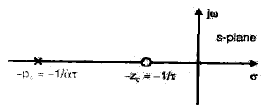• It has a zero at s = - 1/τ and a pole at s = - 1/τ with the zero closer to the origin than the pole.
• The general form of the lead compensator is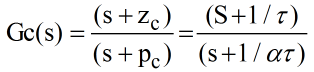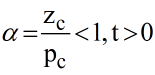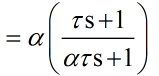• The lead compensator with the transfer function can be realized by an electric lead network shown in figure (a).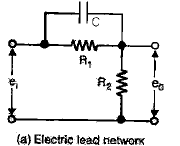LAG COMPENSATOR

• The s-plane representation of lag compensator is shown in figure below: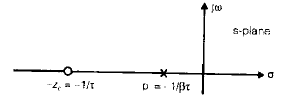• It has a pole at –1/βτ and a zero at -1/τ with the zero located to the left of the pole on the negative real axis. The general form of the transfer function of the lag compensator is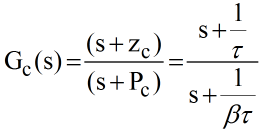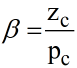• The realization of the transfer function of equation (9.9) is achieved with an electric lag network shown is figure below.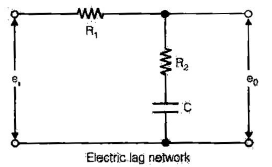From which we can writem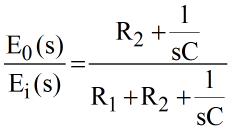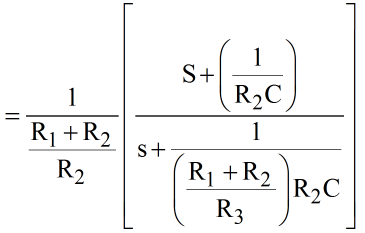Comparing equation (9.9) and (9.10), we get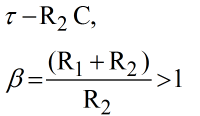therefore, the transfer function of the network becomes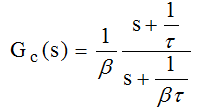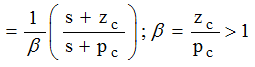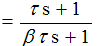• It is to be notes that compared to the form of transfer function (9.11) Gc(s) realized by the network has a multiplicative factor of 1/β. As in the case of lead network realization, we have an additional degree of freedom in the lag network realization also, which is used for impedance matching.
• The sinusoidal transfer function of the lag network is given by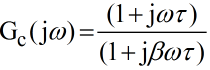Since β >1, the steady state output has a lagging phase angle with respect to the sinusoidal input and hence the name lag network.

• The Bode diagram of the lag network is drawn in figure. The maximum phase lag Øm and the corresponding frequency wm are obtained from equation (9.7) and (9.6) respectively by replacing α with β.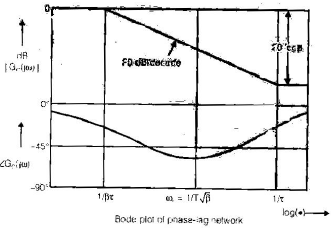• From figure it is observed that the lag network has a d.c. gain of unity while it offers a high frequency gain of 1/β. Since β >1, it means that the high frequency noise is attenuated in passing through the network there by the signal to noise ratio is improved, in contrast to the lead network a typical choice of β is 10.

• The lag-lead compensator is a combination of a lag compensator and a lead compensator. The lag-section has one real pole and one real zero with the pole to the right of zero. The lead-section also has one real pole and real zero but the zero is to the right of the pole.
• The general form of this compensator is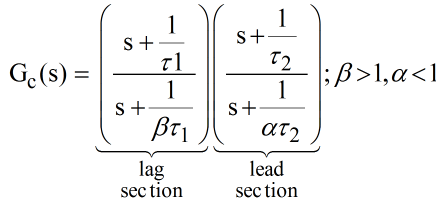• The equation (9.14) can be realized by a single electric lag-lead network shown in figure.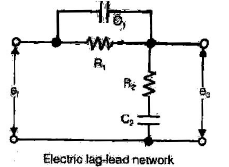• From this figure, the transfer function of the network is given by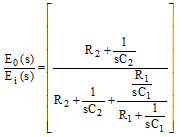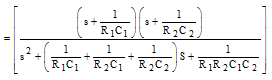•  Comparing equation (9.14) and (9.15), we have

R1 C1 = τ1

R2C= τ2

R1R2C1C2 = αβττ2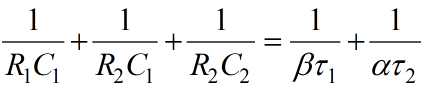From equations (9.16), (9.17) and (9.18), it is found that,. αβ = 1

• It means that a single lag -lead network does not permit us an independent choice of α and β.  Keeping this in view, the transfer function of laglead compensator may be written as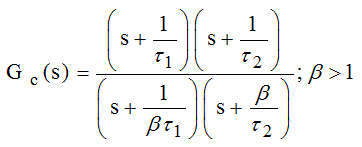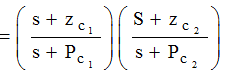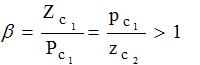where ,τ1 = R1C1, τ= R2C2 and β > 1 such that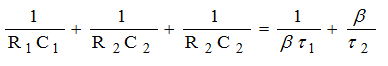• The s-plane representation of a lag lead compensator is shown in figure.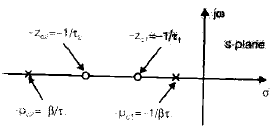• The sinusoidal transfer function of lag-lead compensator is given by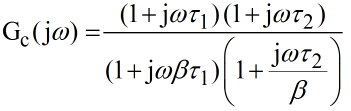• The corresponding Bode plot is given in figure below: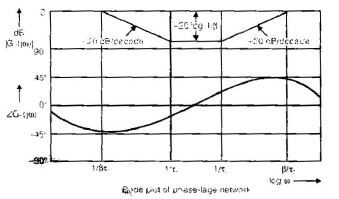• Improves the transient response
• Increases the margin of stability that means improves stability.
• Increases the bandwidth
• The lowest level in output increases. (S/N) output < (S/N) input
• It helps to increase error constant up to some extent.

EFFECTS OF LAG COMPENSATOR

• If effects of lag compensator and lead is to be obtained simultaneously we use lag-lead compensator.
• Lag-lead network introduces both steady state and transient response improvement of the system.

Note: For lead lag compensator the position of compensating poles and zeros of lag lead compensator can be interchanged.

Basic Control Actions & Industrial Automatic Controllers

• As automatic controller compares the actual value of the plant output with the reference input (desired value), it determines the deviation, and produces a control signal that will reduce the deviation to zero or to a small value. The manner in which the automatic controller produces the control signal is called the control action.

Proportional Controllers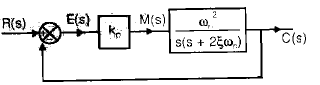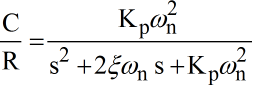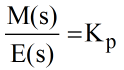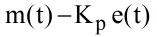Characteristic equation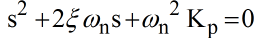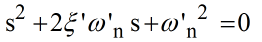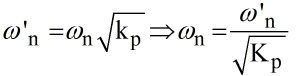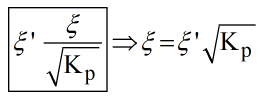Effects: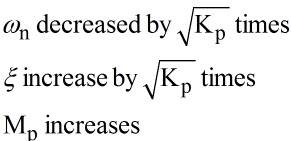Derivative Controllers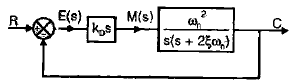KD-Controller Parameter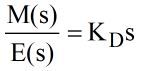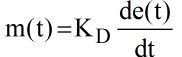Note: The output of controller can be predicted on the basis of knowledge of the slope of error.

∴ derivative controller is anticipatory in nature giving output in advance.

Effects:

• Type and order of the system reduces by one.
• Oscillations has died out hence transient response improves.
• Not used in isolation.

PD Controller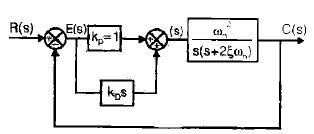Note: PD controller is anticipatory because it is having derivative action also.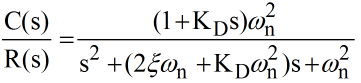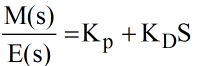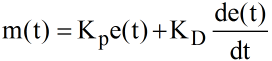• Comparing the characteristic equation with standard one.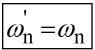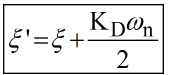Effect:

• Transient response improves
• Damping ratio increases
• Peak overshoot decreases
• BW increases
• System becomes more prone to noise means noise level increases
• Improves gain margin, phase margin, peak resonant.

Pl controller (memory based)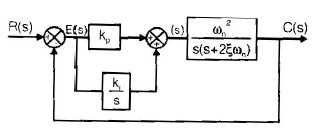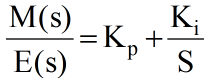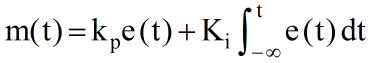Effect:

• Improves type and order of system by one
• Noise level is reduced
• BW reduces
• Increases error constant.
Offer running on EduRev: Apply code STAYHOME200 to get INR 200 off on our premium plan EduRev Infinity!

65 docs|37 tests

,

,

,

,

,

,

,

,

,

,

,

,

,

,

,

,

,

,

,

,

,

,

,

,

,

,

,

;